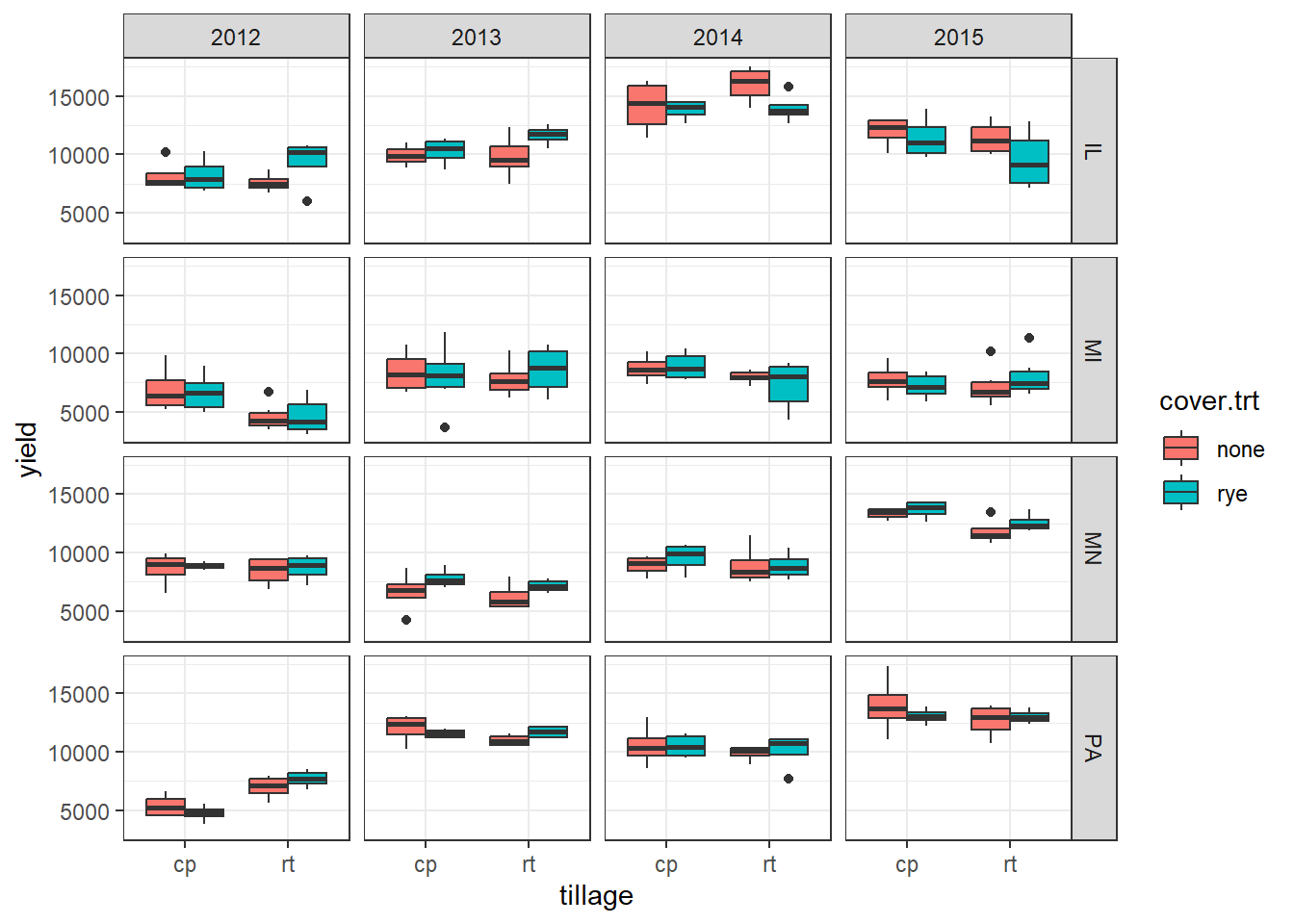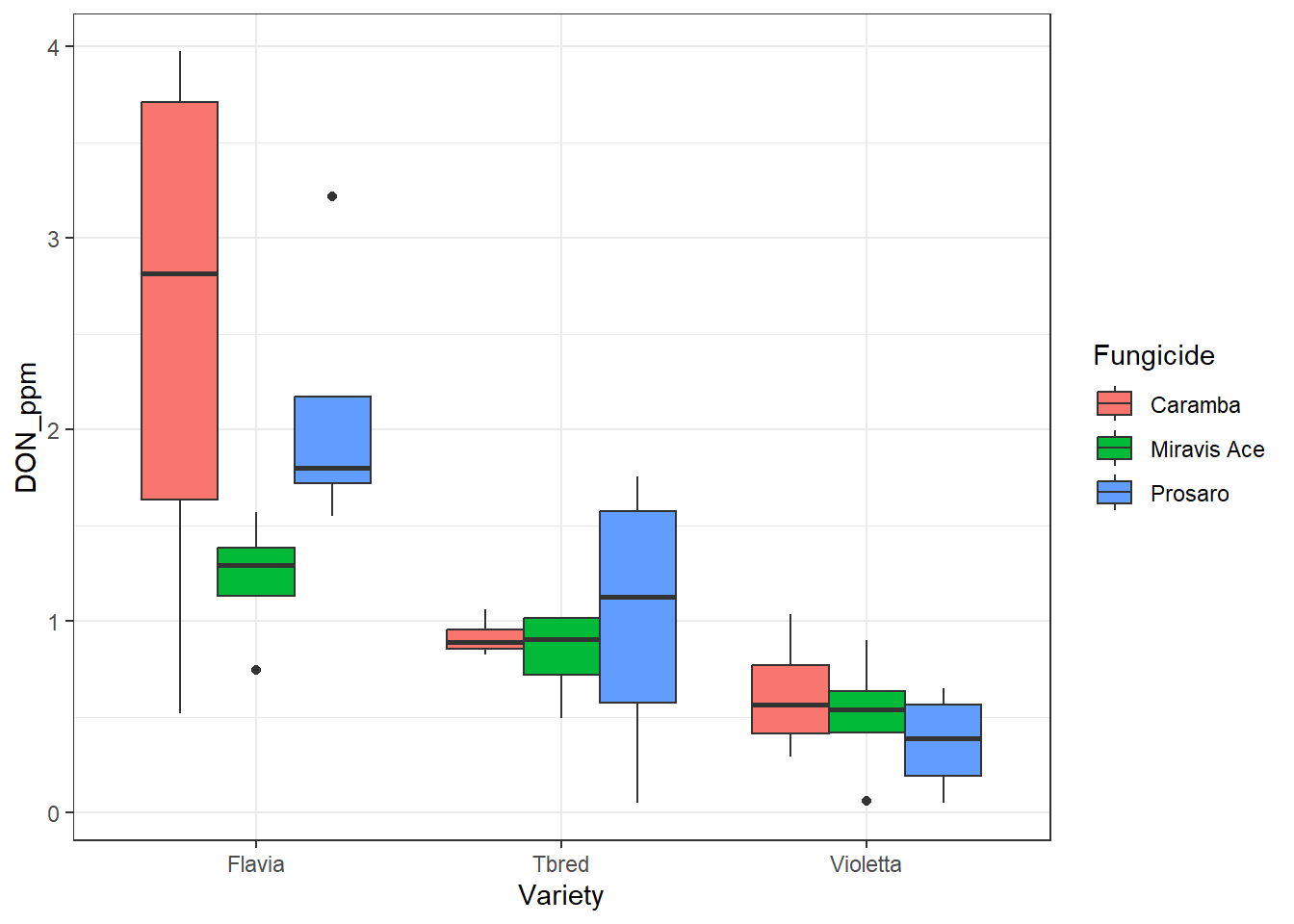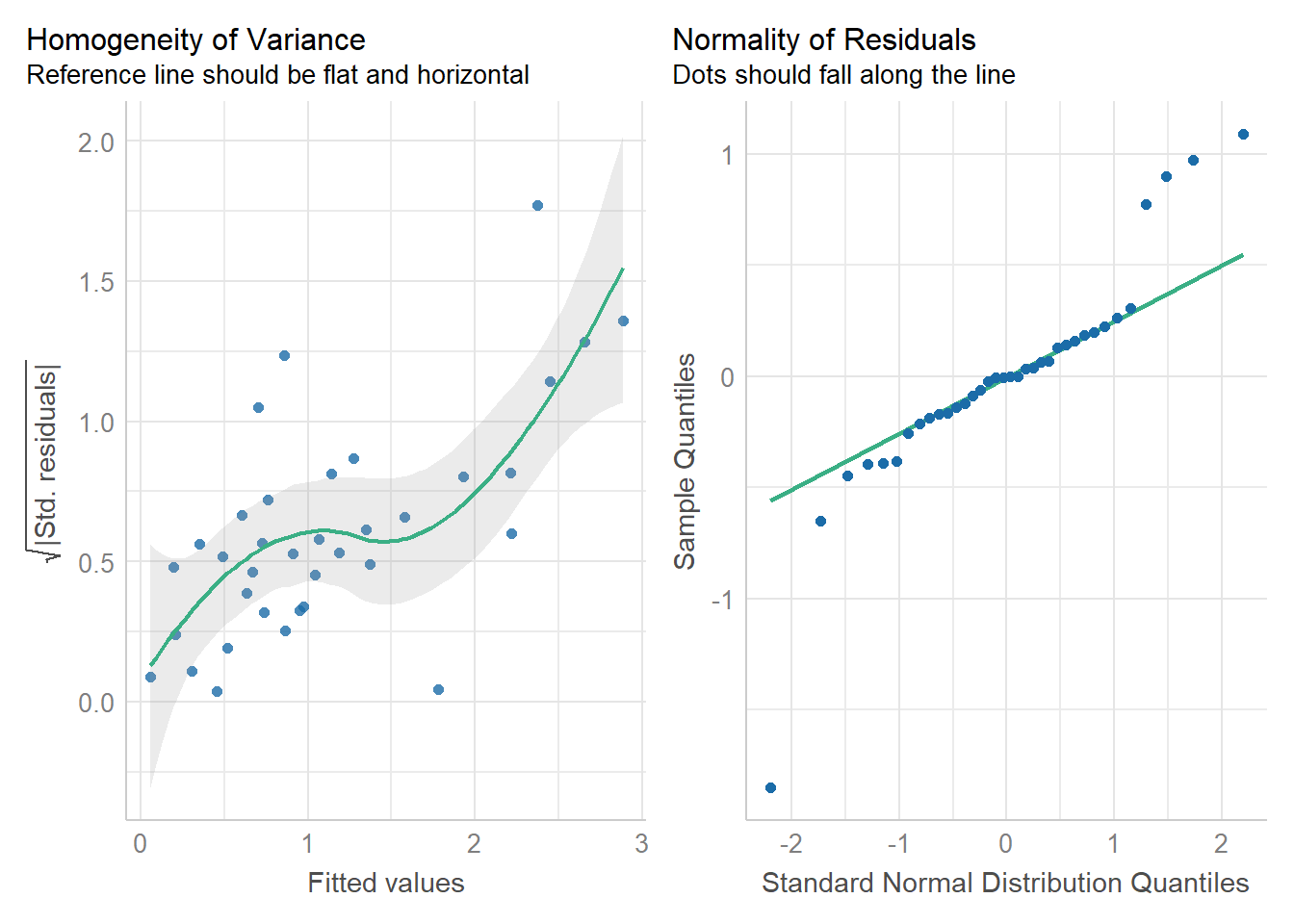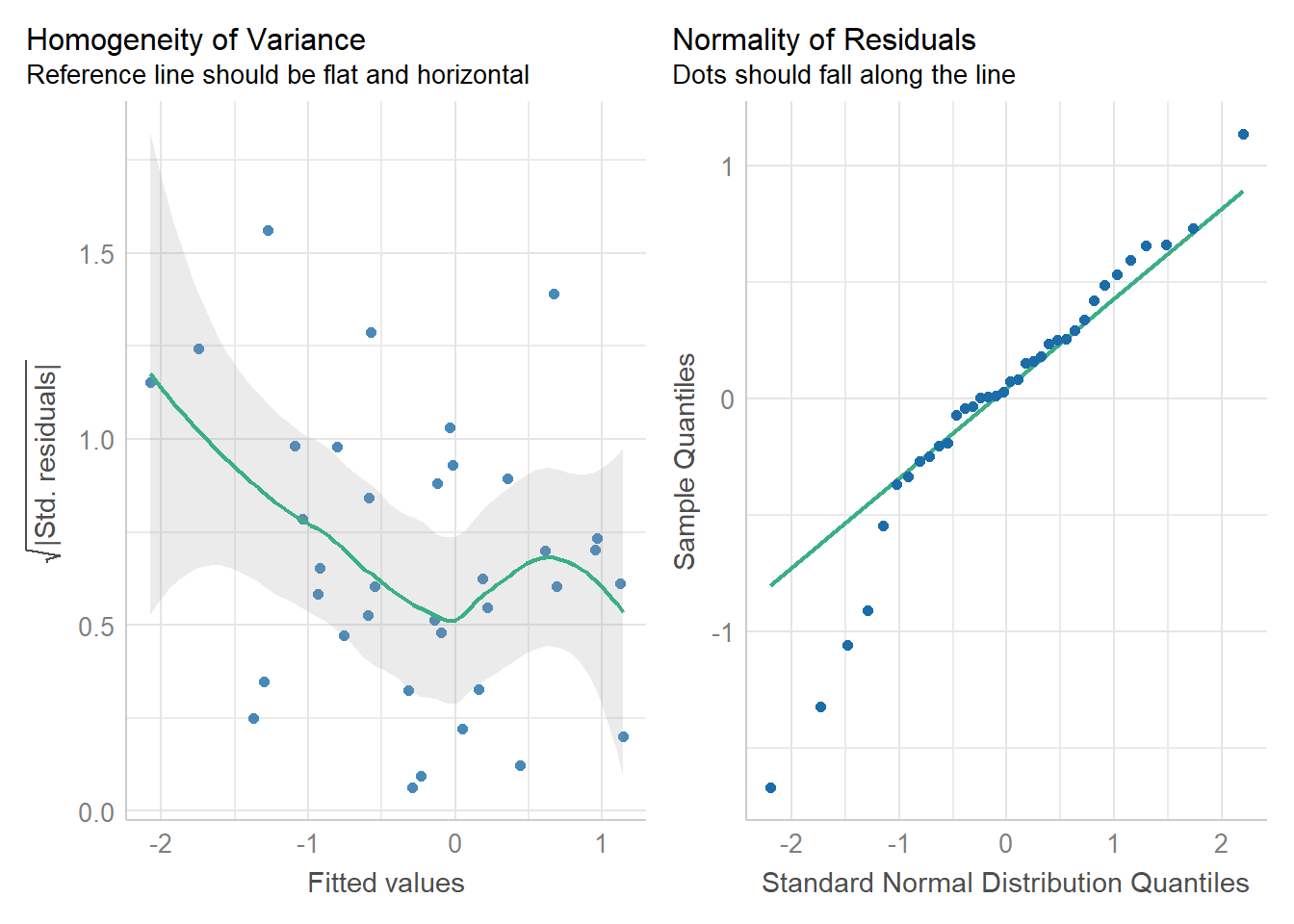# Lesson 4 learning objectives

At the end of this lesson, students will …

• Fit a linear mixed model with crossed and nested random effects.
• Fit a linear mixed model with a transformed response variable.

# Crossed and nested random effects

For this next example, we are finally going to use some real data! We’re going to use a dataset with results from a study of Precision Zonal Management (PZM) conservation agriculture practices (data hosted on Ag Data Commons). In this study, the experimental treatments were two different tillage systems crossed with presence or absence of rye cover crop. The original dataset contains results for both maize and soybean yields but I am giving you only the maize yield data for simplicity.

library(tidyverse)
library(easystats)
library(lme4)
library(lmerTest)
maize <- read_csv('https://usda-ree-ars.github.io/SEAStats/mixed_models_in_R/datasets/pzm_maize.csv')
## Rows: 288 Columns: 8
## ── Column specification ────────────────────────────────────────────────────────
## Delimiter: ","
## chr (4): location, crop, tillage, cover.trt
## dbl (4): yr, plot, block, yield
##
## ℹ Use spec() to retrieve the full column specification for this data.
## ℹ Specify the column types or set show_col_types = FALSE to quiet this message.

Take a look at the data frame.

glimpse(maize)
## Rows: 288
## Columns: 8
## $location <chr> "IL", "IL", "IL", "IL", "IL", "IL", "IL", "IL", "IL", "IL", … ##$ yr        <dbl> 2012, 2012, 2012, 2012, 2012, 2012, 2012, 2012, 2012, 2012, …
## $crop <chr> "m", "m", "m", "m", "m", "m", "m", "m", "m", "m", "m", "m", … ##$ plot      <dbl> 101, 102, 103, 104, 203, 204, 207, 208, 301, 302, 305, 306, …
## $block <dbl> 1, 1, 1, 1, 2, 2, 2, 2, 3, 3, 3, 3, 4, 4, 4, 4, 1, 1, 1, 1, … ##$ tillage   <chr> "rt", "cp", "rt", "cp", "rt", "cp", "cp", "rt", "cp", "rt", …
## $cover.trt <chr> "rye", "rye", "none", "none", "rye", "rye", "none", "none", … ##$ yield     <dbl> 10784, 8552, 8728, 10194, 9946, 6882, 7388, 7276, 10230, 104…

There are multiple locations and multiple years. The locations are identified by state. The plot and block column have integers identifying the plots and blocks. The tillage column has two different values, "cp" (chisel plow) and "rt" (ridge tillage). The cover.trt column also has two different values, "none" and "rye".

We need to convert the yr, plot, and block columns to factor type because they are integers and we want to treat them as categorical for the model. We will use mutate() to do this.

maize <- maize %>%
mutate(
yr = factor(yr),
plot = factor(plot),
block = factor(block)
)

PROTIP: If you give your plot and block columns character ID’s, not numeric ID’s, to begin with (such as P1, P2, etc.), you will not have to worry about them accidentally being considered numeric variables in your models.

Make boxplots of the yield data using geom_boxplot(). Tillage treatment is on the x axis, cover treatment is distinguished by color of the boxes, and yield is on the y axis. We use facet_grid() to make a separate plot for each combination of location and year.

ggplot(maize, aes(y = yield, x = tillage, fill = cover.trt)) +
facet_grid(location ~ yr) +
geom_boxplot(position = position_dodge()) +
theme_bw()This is a fairly complicated situation that is typical of multi-location multi-year trials. There are some location-year combinations in which the tillage treatments seem to result in different yields, and mostly minimal differences between the cover treatments. However the majority of the variation is between locations and years.

How do we set up this model? Well, we definitely want to have a fixed effect for tillage treatment, and another for cover treatment, and the interaction between the two. So our starting point is:

yield ~ tillage + cover.trt + tillage:cover.trt

The next question is, should location and year be random or fixed? This depends on the statistical inference we want to draw. If we are interested in change over time, year should be a fixed effect. But if we think of the years the study was conducted as a “random sample” of the potential years we could have used, and we are not interested in comparing means of the different years, year should be a random effect. The same goes for location. For the sake of this example, let’s say year and location will both be random effects. We will fit them as crossed random effects, meaning an intercept will be fit for each year and each location. This means we assume the effect of year is constant across locations, and vice versa. So now we have

yield ~ tillage + cover.trt + tillage:cover.trt + (1 | yr) + (1 | location)

Notice I did not include random slopes, only random intercepts. It could potentially also make sense to include random slopes, if we think the treatment effect is different by location and year, not just that the locations and years have different “baseline” yields.

We also have many blocks at a given location, each of which has many plots. Block should definitely be treated as a random effect nested within location. Block 1 in Illinois is not the same as Block 1 in Minnesota; it would make no sense to have a common intercept for Block 1. Thus block is nested within location, meaning a separate intercept is fit for each block in each location. We do not need to include plot explicitly, because the plots are individual data points. The random variation of plots is already taken care of by the overall model residuals. This will add one more random intercept term to our model. We use the : operator to separate nesting terms.

yield ~ tillage + cover.trt + tillage:cover.trt + (1 | yr) + (1 | location) + (1 | block:location)

Here is the model.

fit_maize <- lmer(yield ~ tillage + cover.trt + tillage:cover.trt + (1 | yr) + (1 | location) + (1 | block:location), data = maize)

An alternative here would be to give each location-year combination its own intercept. This would mean that location is nested within year as well, resulting in three levels of nesting, year : location : block. That might make sense in this case if the locations are far enough apart that we do not think the random variation of years would be consistent across locations. The formula would look like this:

yield ~ tillage + cover.trt + tillage:cover.trt + (1 | location:yr) + (1 | block:location:yr)

If we wanted to do random slopes in this model, we would have to specify which terms would be random and which grouping factors would get separate slopes fitted to them. For example, we might want to fit only random slopes for the main effect terms, and only for the year-location combination. The formula would look like this:

yield ~ tillage + cover.trt + tillage:cover.trt + (tillage + cover.trt | location:yr) + (1 | block:location:yr)

These examples demonstrate how many modeling decisions have to be made for even a relatively clean and neat dataset.

# Data transformations

Typically, researchers think of data transformation as something you only need to do to “make the data normal” (which really means making the residuals roughly normally distributed). This is not really the best approach. Transforming data should be done for scientific reasons, not just on an ad hoc basis. For example, if a response variable is a ratio or a concentration, it typically would make sense to take the logarithm of that value before fitting the statistical model. This is because the difference between two values on a logarithmic scale is multiplicative, not additive. The difference between a ratio of 0.01 and 1 is 100 times, which is the same difference as the difference between a ratio of 1 and 100. That is only true on a logarithmic scale.

Here is an example situation where you might want to consider doing a log transformation. These data are a small subset of an ARS scientist’s data. We have different varieties of barley crossed with different types of fungicide. Multiple plots of each combination of variety and fungicide were grown in an experimental block (called Rep in the dataset). Among other responses, the amount of DON (a mycotoxin) produced was measured, which is given here in units of parts per million (column DON_ppm).

barley_don <- read_csv('https://usda-ree-ars.github.io/SEAStats/mixed_models_in_R/datasets/barley_don.csv')
## Rows: 36 Columns: 7
## ── Column specification ────────────────────────────────────────────────────────
## Delimiter: ","
## chr (3): Rep, Variety, Fungicide
## dbl (4): weight_kg, DON_ppm, yield_bu_ac, PIK
##
## ℹ Use spec() to retrieve the full column specification for this data.
## ℹ Specify the column types or set show_col_types = FALSE to quiet this message.

What do the data look like?

glimpse(barley_don)
## Rows: 36
## Columns: 7
## $Rep <chr> "A", "A", "A", "A", "A", "A", "A", "A", "A", "B", "B", "B"… ##$ Variety     <chr> "Violetta", "Violetta", "Violetta", "Tbred", "Tbred", "Tbr…
## $Fungicide <chr> "Prosaro", "Miravis Ace", "Caramba", "Prosaro", "Miravis A… ##$ weight_kg   <dbl> 1.0754063, 1.4398031, 1.3404487, 2.7349834, 2.7669905, 2.7…
## $DON_ppm <dbl> 0.05042487, 0.06059087, 0.29583135, 0.05234182, 0.49502204… ##$ yield_bu_ac <dbl> 80.94682, 99.71024, 81.44227, 172.83955, 181.55261, 171.69…
## \$ PIK         <dbl> 11, 11, 14, 10, 32, 32, 35, 18, 24, 23, 23, 23, 46, 46, 45…
ggplot(barley_don, aes(x = Variety, y = DON_ppm, fill = Fungicide)) +
geom_boxplot(position = position_dodge()) +
theme_bw()We can fit a fairly simple model to these data, with variety, fungicide type, and their interaction as fixed effects, and experimental block (Rep) as random intercept.

fit_don <- lmer(DON_ppm ~ Variety + Fungicide + Variety:Fungicide + (1|Rep), data = barley_don)

However, I would recommend fitting a model with the log-transformed DON_ppm variable as the response variable. You can do the log transformation directly in the model statement by surrounding the name of the variable with log().

fit_don_log <- lmer(log(DON_ppm) ~ Variety + Fungicide + Variety:Fungicide + (1|Rep), data = barley_don)

It makes sense to fit the model to the logarithm of DON concentration because concentration is on a ratio scale, so multiplicative changes in concentration are the most meaningful to consider.

Even though I just said that the rationale behind transforming data should be scientific and not just about making the data behave well, it does help us improve the residuals. In this case we can see that the residuals of the model fit to the untransformed data show very non-homogeneous variance and many points that far fall off the normal Q-Q plot. The situation is much improved for the model fit to the log-transformed data.

Notice we’re using the check_model() function from easystats but with the check argument to tell it to produce only the two diagnostic plots we are interested in. Look at the help documentation for ?check_model to see all the options.

check_model(fit_don, check = c('homogeneity', 'qq'))
## Model has interaction terms. VIFs might be inflated.
##   You may check multicollinearity among predictors of a model without
##   interaction terms.check_model(fit_don_log, check = c('homogeneity', 'qq'))
## Model has interaction terms. VIFs might be inflated.
##   You may check multicollinearity among predictors of a model without
##   interaction terms.We will revisit this model in a later lesson when we talk about comparing means.

# Exercises

For Exercise 1 and 2, we will use the fisher.barley dataset which is part of the agridat package, an R package that has a bunch of classic datasets from agricultural science. You can load the dataset using

data(fisher.barley, package = 'agridat')

NOTE: If you are running R locally and were unable to install the agridat package, I have provided the dataset as a CSV file: fisher.barley <- read_csv('https://usda-ree-ars.github.io/SEAStats/mixed_models_in_R/datasets/fisher.barley.csv')

### Exercise 1

Examine the dataset. We have five genotypes of barley (gen) being grown across six environments in Minnesota (env) in two different years (year): 1931 and 1932.

Convert year to a factor varaible in the data frame. Why is it important to do this?

• Hint: Use mutate() and factor().

### Exercise 2

1. Fit a linear mixed model with yield as a response variable, genotype gen as a fixed effect, and environment env and year as crossed random intercepts.
• Hint: (1|env) and (1|year)
1. Fit a linear mixed model with the same response variable and fixed effect, but include environment and year nested within environment as random intercepts.
• Hint: You will need to incorporate year:env into the new formula.
1. What is the difference, if any, between the models’ results?
• Hint: Try summary(), fixef(), ranef(), and anova().

### Exercise 3

For exercises 3 and 4, we will use data collected by ARS scientists (with details removed and random noise added). The data come from a bioassay where bacterial biomass was measured in an experimental treatment with the goal of reducing biomass, and compared with two different negative controls. The scientists did four runs of the growth assay, with variable numbers of replicates in each run. THe response variable is biomass which must be a positive value.

bioassay <- read_csv('https://usda-ree-ars.github.io/SEAStats/mixed_models_in_R/datasets/bioassay.csv')
1. Fit a linear mixed model with biomass as the response variable, treatment as a fixed effect, and experiment as a random intercept. What do you notice?